# plotnineplotnine is an implementation of a grammar of graphics in Python, it is based on ggplot2. The grammar allows users to compose plots by explicitly mapping data to the visual objects that make up the plot.

Plotting with a grammar is powerful, it makes custom (and otherwise complex) plots easy to think about and then create, while the simple plots remain simple.

To find out about all building blocks that you can use to create a plot, check out the documentation. Since plotnine has an API similar to ggplot2, where we lack in coverage the ggplot2 documentation may be of some help.

## Example

```from plotnine import *
from plotnine.data import mtcars```

Building a complex plot piece by piece.

1. Scatter plot

```(ggplot(mtcars, aes('wt', 'mpg'))
+ geom_point())```
2. Scatter plot colored according some variable

```(ggplot(mtcars, aes('wt', 'mpg', color='factor(gear)'))
+ geom_point())```
3. Scatter plot colored according some variable and smoothed with a linear model with confidence intervals.

```(ggplot(mtcars, aes('wt', 'mpg', color='factor(gear)'))
+ geom_point()
+ stat_smooth(method='lm'))```
4. Scatter plot colored according some variable, smoothed with a linear model with confidence intervals and plotted on separate panels.

```(ggplot(mtcars, aes('wt', 'mpg', color='factor(gear)'))
+ geom_point()
+ stat_smooth(method='lm')
+ facet_wrap('~gear'))```
5. Make it playful

```(ggplot(mtcars, aes('wt', 'mpg', color='factor(gear)'))
+ geom_point()
+ stat_smooth(method='lm')
+ facet_wrap('~gear')
+ theme_xkcd())```

## Installation

Official release

```# Using pip
\$ pip install plotnine         # 1. should be sufficient for most
\$ pip install 'plotnine[all]'  # 2. includes extra/optional packages

# Or using conda
\$ conda install -c conda-forge plotnine```

Development version

`\$ pip install git+https://github.com/has2k1/plotnine.git`

## Contributing

Our documentation could use some examples, but we are looking for something a little bit special. We have two criteria:

1. Simple looking plots that otherwise require a trick or two.
2. Plots that are part of a data analytic narrative. That is, they provide some form of clarity showing off the geom, stat, ... at their differential best.

If you come up with something that meets those criteria, we would love to see it. See plotnine-examples.

If you discover a bug checkout the issues if it has not been reported, yet please file an issue.

And if you can fix a bug, your contribution is welcome.

###### Hassan KibirigeWe are re-licensing plotnine to MIT. It was only licensed as GPL since it was derived from ggplot2 which was GPL at the time, and, now that ggplot2 is MIT, we can relicense plotnine.

To make this change to plotnine, we need the approval of all copyright holders, which I have found by reviewing contributions from all contributors made after the January 2017 change to GPLv2.

@akdor1154, @Alyetama, @astrocorgi, @CarlosGrohmann, @ChickenProp, @dulacp, @Fischmiep, @gokceneraslan, @hugovk, @hyiltiz, @JarnoRFB, @jdanbrown, @jeroenjanssens, @joshhartmann11, @jsoma, @jsspencer, @khaeru, @kingishb, @kngwyu, @machow, @stillmatic, @stonebig, @tabedzki, @thomasjpfan, @tr8dr, @TyberiusPrime, @yejianye, would you permit us to re-license plotnine with the MIT license? If so, please comment "I agree" below.

This pull requests adds 'annotation_logticks()' similar to the ggplot2 one: https://ggplot2.tidyverse.org/reference/annotation_logticks.html

This adds log-10 'log ticks' as an annotation similar to a rug to the plot.

Looks like this: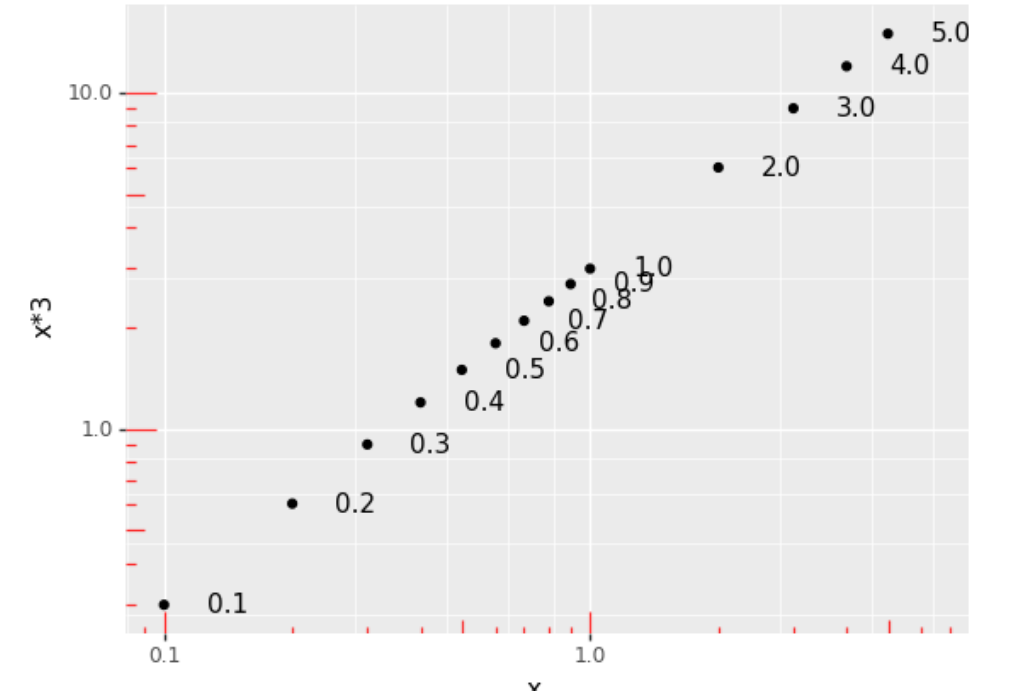Example usage:

``````import pandas as pd
import plotnine
df = pd.DataFrame({"x": [0.1, 0.2, 0.3, 0.4, 0.5, 0.6, 0.7, 0.8, 0.9, 1.0,
2, 3, 4, 5]})
p = plotnine.ggplot(df, plotnine.aes('x','x*3'))
p += plotnine.geom_point()
p += plotnine.geom_text(plotnine.aes(label='x'),
ha='left', nudge_x=.1)
p += plotnine.scale_y_log10()
p += plotnine.scale_x_log10()
#p += plotnine.geom_rug(sides='lb', width=2, alpha=0.5) #  to check correctness
p += plotnine.geoms.annotation_logticks(sides='lb', color='red',
alpha=0.8, width=(1.2,.75, 0.4),
linetype='solid')

p
``````

Right now this is not perfect. For one, it's a 'fake geom' that ignores it's data.

And I've failed to detect when the axis are not log10, and it then will plot misleading tick marks. Can't for the life of me figure out how the geom could detect it though - coord.is_linear is always true.

• #### input validation on geom_violin(draw_quantiles)

This PR checks, normalizes and documents the draw_quantiles parameter on geom_violin.

It now accepts any iterable of floats, or a single float (to be feature compatible with ggplot2). They get converted into a list internally.

If it's not valid (ie. not float, [float] or values outside of 0..1 (exclusive), a ValueError is raised when calling geom_violin.

Previously, the following happened:

• draw_quantiles = True -> Exception in drawing `TypeError: object of type 'bool' has no len()`
• draw_quantiles = 0.5 -> Exception in drawing: `TypeError: object of type 'float' has no len()`
• draw_quantiles = np.array([0.5]) -> ok
• draw_quantiles = np.array([0.3, 0.5]) -> exception in drawing: `ValueError: The truth value of an array with more than one element is ambiguous. Use a.any() or a.all()`
• draw_quantiles = 0 -> interpreted as False
• draw_quantiles = [0.0] -> exception in drawing: `ValueError: A value in x_new is below the interpolation range.`
• draw_quantiles = [1.0] -> exception in drawing: `ValueError: A value in x_new is above the interpolation range.`
• draw_quantiles = [1.25] -> exception in drawing: `ValueError: A value in x_new is above the interpolation range.`

These all now either work, or throw a sensible exception `ValueError("draw_quantiles must be a float or an iterable of floats (>0.0; < 1.0)")` much closer to the actual erroneous call.

• #### Use aliased imports in the gallery

I would like to suggest using "import plotnine as p9" (or something like that) in the gallery code instead of "from plotnine import *", and then using "p9" as a prefix for all identifiers defined by plotnine. That would make it much clearer which of them come from plotnine and which don't.

• #### introduced annotation_alternating_backgrounds

This PR introduces an annotation that adds 'alternating' backgrounds, which is useful to increase readability in tight jitter plots on categorical x-axis data. Example:

``````import plotnine as p9
from plotnine.data import mtcars
g = p9.ggplot(mtcars.assign(
gear = pd.Categorical(mtcars.gear),
am = pd.Categorical(mtcars.am)
))
g += p9.geom_jitter(p9.aes("gear", 'wt', color='am'), width=.45, height=0)
g += p9.annotation_alternating_background(fills=['grey','darkgrey'],
alpha=.5)
g += p9.scale_color_manual(['red','blue'])
``````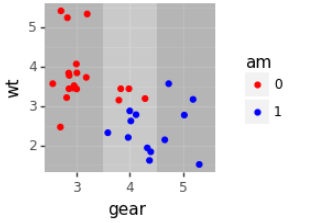Supports coord_flip: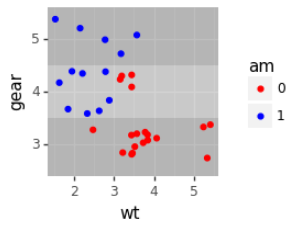And more than 2 colors, and does something sensible with non-discrete axis (ie. change shade halfway between labels)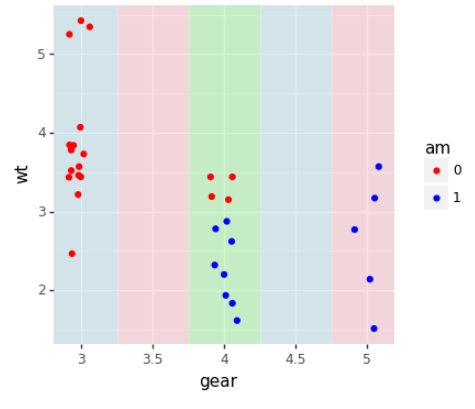Here's a first implementation of adust_text using a position - see #218 I have extended the position to allow a post-drawing callback, and positions can now define a list of geoms that they work with.

While this enables the basic use case, there are some kinks we should probably talk with upstream about:

``````(p9.ggplot(mtcars, p9.aes('mpg','disp')) + p9.geom_point()
+ p9.geom_text(p9.aes(label='name'), color='blue', position = p9.position_adjust_text(), size=7)
)
``````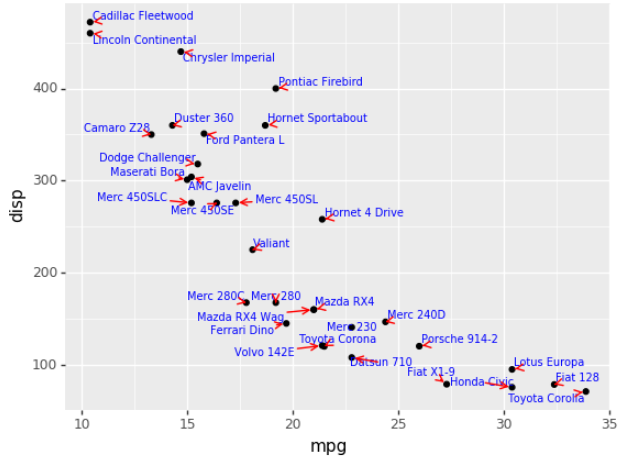1. Two sets of points and labels don't work well:
``````g = (p9.ggplot(mtcars, p9.aes('mpg','disp'))
+ p9.geom_point()
+ p9.geom_text(p9.aes(label='name'), color='blue',
'arrowprops': {'arrowstyle': '-', 'color': 'blue'}
}), size=7)
+ p9.geom_point(p9.aes('mpg+1','disp'), color='green')
+ p9.geom_text(p9.aes(x='mpg+1', label='name'), color='green',
'arrowprops': {'arrowstyle': '-', 'color': 'green'}
}), size=7)
)
g
``````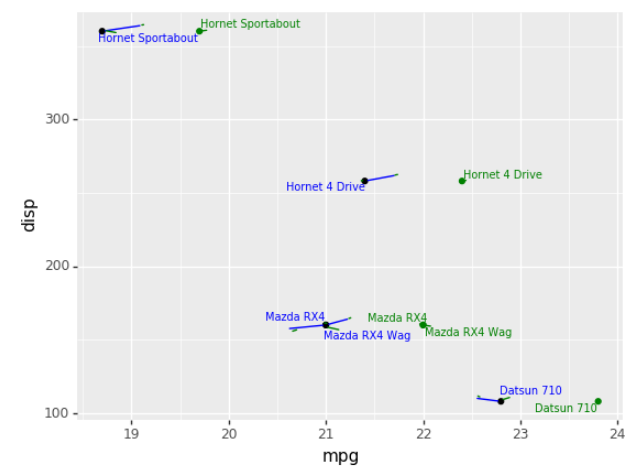(I think it's an issue with both set's of text labels being drawn on the same ax, and accordingly the second call sees the first set again. Will have to debug.)

1. labels and arrows interact badly
``````(p9.ggplot(mtcars, p9.aes('mpg','disp')) + p9.geom_point()
+ p9.geom_label(p9.aes(label='name'), color='blue', position = p9.position_adjust_text(), size=7)
)
``````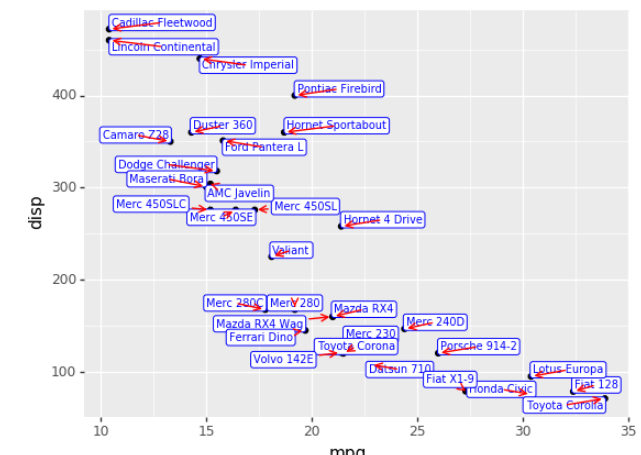1. no geom_point() -> broken plot
``````(p9.ggplot(mtcars, p9.aes('mpg','disp'))
# + p9.geom_point()
+ p9.geom_text(p9.aes(label='name'), color='blue', position = p9.position_adjust_text(), size=7)
)
``````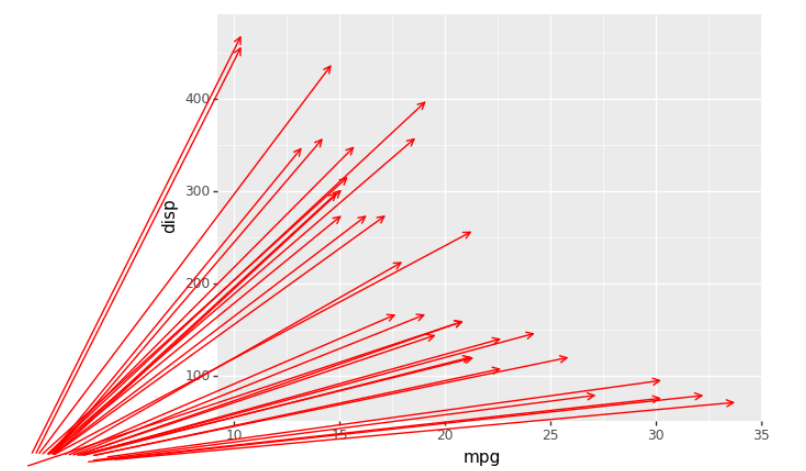3b) geom_point() after geom_text(position_adjust_text) > broken plot

``````(p9.ggplot(mtcars, p9.aes('mpg','disp'))
+ p9.geom_text(p9.aes(label='name'), color='blue', position = p9.position_adjust_text(), size=7)
+ p9.geom_point()
)
``````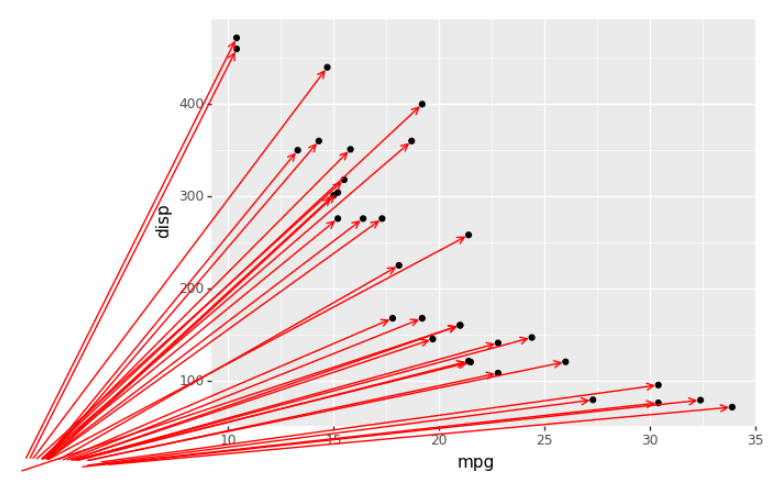Also, I note that the adjustment is not fast - it takes a few seconds ever for these 'simple' plots.

• #### New matplotlib warnings - when using plotnine 0.4.0

I am getting some new warnings when using plotnine. My point is - let's make sure, plotnine adapts to the latest matplotlib changes.

USED VERSIONS & PLATFORMS: OS: Win10 64-bit Anaconda Python: 3.6.6

matplotlib.version '3.0.0' plotnine.version '0.4.0'

WARNINGS EXAMPLES: C:\Users\stesim\Anaconda3\envs\py36\lib\site-packages\plotnine\coords\coord_cartesian.py:31: MatplotlibDeprecationWarning: The Bunch class was deprecated in Matplotlib 3.0 and will be removed in 3.2. Use types.SimpleNamespace instead. self.limits = Bunch(xlim=xlim, ylim=ylim)

C:\Users\stesim\Anaconda3\envs\py36\lib\site-packages\plotnine\facets\layout.py:147: MatplotlibDeprecationWarning: The Bunch class was deprecated in Matplotlib 3.0 and will be removed in 3.2. Use types.SimpleNamespace instead. return Bunch(x=xsc, y=ysc)

C:\Users\stesim\Anaconda3\envs\py36\lib\site-packages\plotnine\coords\coord.py:144: MatplotlibDeprecationWarning: The Bunch class was deprecated in Matplotlib 3.0 and will be removed in 3.2. Use types.SimpleNamespace instead. y=panel_params['y_range'])

• #### Allow user to change relative width or height of facets

This change allows a user of facet_grid() to specify an optional:

• space={'y': `<ratios>`}: setting the relative height of vertical facets
• for example facet_grid("pane ~ .", scales='free_y', space={'y': [2,1,1]}. This would make the top facet height 2x that of the 2nd and 3rd facet
• space={'x': `<ratios>`}: setting the relative width of horizontal facets
• for example space={'x': [1,2,1], ...}
• or a combination of the two:
• space={'x': [1,2,1], 'y': [2,1,1]}.

A similar extension exists for R ggplot(), though not in the main ggplot package. Here is an example:

``````import numpy as np
import pandas as pd
import plotnine
from plotnine import *

y = pd.Series(np.random.normal(0.0, 1.0, 400)).cumsum()
df = y.diff().fillna(0.0)
x = np.arange(0,400)

gdf = pd.concat([
pd.DataFrame({'x': x, 'y': y, 'what': 'y', 'pane': 'cumr'}),
pd.DataFrame({'x': x, 'y': df, 'what': 'df', 'pane': 'df'}),
])

(ggplot() +
geom_line(aes(x='x', y='y', color='what'), data=gdf) +
facet_grid("pane ~ .", scales="free_y", space={'y': [3,1]}))
``````

See the attached output included.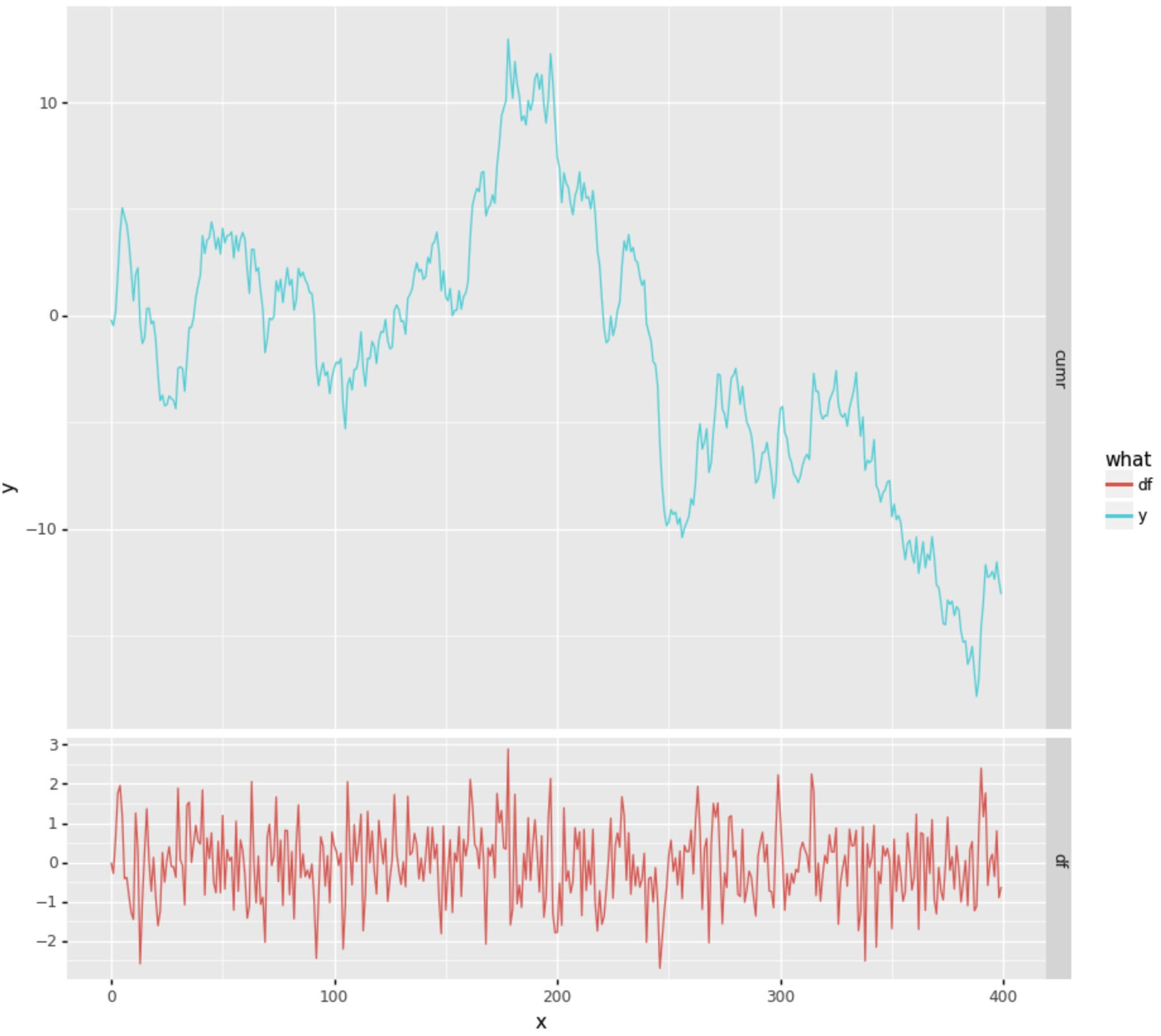• #### Plotnine warnings cherry

This PR introduces a new Warning class PlotNineWarning, which is now used throughout the plotnine code base when emitting warnings

It allows the downstream to capture PlotNine warnings in a structured way.

Note that PlotNine will still emit UserWarnings from upstream - they are not being rewritten.

I've also promoted PlotNineException and PlotNineWarning to top-level objects on the module, so they're visible to the user.

• #### plotnine and pyqt5

Hello,

`plotnine` is a wonderful package. I am trying to figure out how to incorporate plots into a `pyqt5` application. For `matplotlib`, there are a number of examples, but with `plotnine`, I don't know where to start. Any guidance is greatly appreciated.

Thank you.

• #### Possible to hide legend?

R ggplot supports `theme(legend.position = "none")`, but plotnine doesn't appear to recognize `'none'` in `theme(legend_position='none')`.

• http://ggplot2.tidyverse.org/reference/theme.html

Is there another way to do this, or is this a feature that would need to be added?

• #### Polars support

Polars https://www.pola.rs/ is a nice data frame library for python.

It would be great if plotnine would also support polars instead of pandas data frames.

My current workaround is:

``````plotnine.ggplot(polars_df.to_pandas(), plotnine.aes('...'))
``````
• #### `axis_ticks` in `theme` not changing color

I can't get the theme element `axis_ticks` to change the color of the ticks. For example, the following code allows me to change the size of the ticks but not the color. I've tried with both the `color` and `colour` arguments. I'm using `plotnine` version 0.8.0. The following code reproduces the issue for me.

``````import plotnine as p9

# panel gridlines are red and with width 3 (good)
# axis ticks change to width 3 but the color remains black
(p9.ggplot() +
p9.geom_point(p9.aes(x = 1, y = 1), size =5) +
p9.theme(axis_ticks = p9.element_line(color = 'red', size = 3),
panel_grid_major = p9.element_line(color = 'red', size = 3)))
``````

Thanks for the support. The `plotnine` package has made my transition from R to python so much easier.

• #### save background

Hi,

The following code save the transparent background. p = ggplot(res,aes(x='date',y='Price',fill='Company'))+geom_tile(aes(width=0.95,height=0.95)) p.save("market.pdf")

How to set the backgound in white color ?

• #### axis labels in mathtext

Hi, I use `geom_line` and `scale_x_log10` to plot my data in log10 along xaxis, the labels are in scientific notation shown like "1e5", however, I would like the label to be shown as \$10^5\$.

In matploblib I can use `rcParams["axes.formatter.use_mathtext"]=True` to achieve that. I tried `theme_matplotlib` with `theme_matplotlib({'axes.formatter.use_mathtext':True})` but it did not work. Is there a way to do that in plotnine?

• #### Area chart with gradient

Is there any way to achieve a gradient in the filling of an area chart?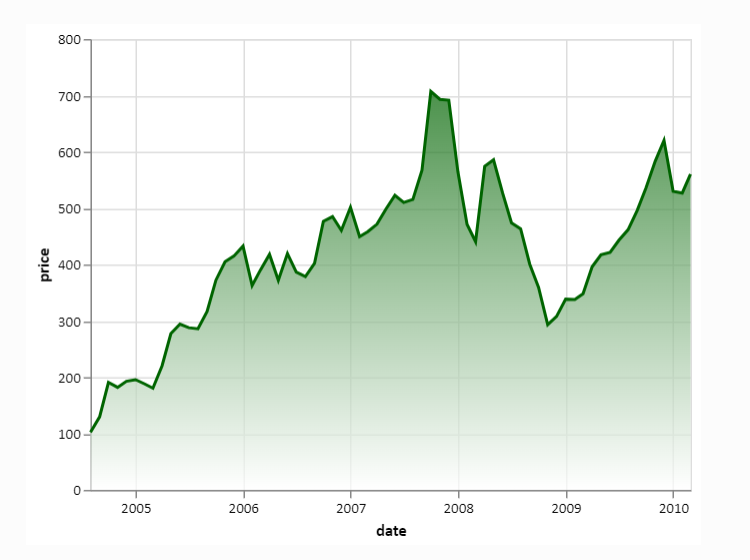I have not been able to find anything in the docs.

If not, how feasible would it be to add this feature? I think it greatly improves the aesthetics of area charts.

###### A grammar of graphics for Python

plotnine Latest Release License DOI Build Status Coverage Documentation plotnine is an implementation of a grammar of graphics in Python, it is based

Feb 18, 2021
###### Simple plotting for Python. Python wrapper for D3xter - render charts in the browser with simple Python syntax.

PyDexter Simple plotting for Python. Python wrapper for D3xter - render charts in the browser with simple Python syntax. Setup \$ pip install PyDexter

Mar 6, 2021
###### Visual Python is a GUI-based Python code generator, developed on the Jupyter Notebook environment as an extension.

Visual Python is a GUI-based Python code generator, developed on the Jupyter Notebook environment as an extension.

Jun 24, 2022
###### A Python Binder that merge 2 files with any extension by creating a new python file and compiling it to exe which runs both payloads.

Update ! ANONFILE MIGHT NOT WORK ! About A Python Binder that merge 2 files with any extension by creating a new python file and compiling it to exe w

Jun 23, 2022
###### Declarative statistical visualization library for Python

Altair http://altair-viz.github.io Altair is a declarative statistical visualization library for Python. With Altair, you can spend more time understa

Jun 28, 2022
###### Interactive Data Visualization in the browser, from Python

Bokeh is an interactive visualization library for modern web browsers. It provides elegant, concise construction of versatile graphics, and affords hi

Jul 1, 2022
###### Cartopy - a cartographic python library with matplotlib support

Cartopy is a Python package designed to make drawing maps for data analysis and visualisation easy. Table of contents Overview Get in touch License an

Jun 28, 2022
###### a plottling library for python, based on D3

Hello August 2013 Hello! Maybe you're looking for a nice Python interface to build interactive, javascript based plots that look as nice as all those

Jul 5, 2022
###### UNMAINTAINED! Renders beautiful SVG maps in Python.

Kartograph is not maintained anymore As you probably already guessed from the commit history in this repo, Kartograph.py is not maintained, which mean

Jun 16, 2022
###### Tools for writing, submitting, debugging, and monitoring Storm topologies in pure Python

Petrel Tools for writing, submitting, debugging, and monitoring Storm topologies in pure Python. NOTE: The base Storm package provides storm.py, which

Dec 18, 2021
###### The Python ensemble sampling toolkit for affine-invariant MCMC

emcee The Python ensemble sampling toolkit for affine-invariant MCMC emcee is a stable, well tested Python implementation of the affine-invariant ense

Jul 2, 2022
###### The windML framework provides an easy-to-use access to wind data sources within the Python world, building upon numpy, scipy, sklearn, and matplotlib. Renewable Wind Energy, Forecasting, Prediction

windml Build status : The importance of wind in smart grids with a large number of renewable energy resources is increasing. With the growing infrastr

Jun 17, 2022
###### Tools for exploratory data analysis in Python

Dora Exploratory data analysis toolkit for Python. Contents Summary Setup Usage Reading Data & Configuration Cleaning Feature Selection & Extraction V

Jun 15, 2022
###### A Python Library for Self Organizing Map (SOM)

SOMPY A Python Library for Self Organizing Map (SOM) As much as possible, the structure of SOM is similar to somtoolbox in Matlab. It has the followin

Jun 30, 2022
###### :bowtie: Create a dashboard with python!

Installation | Documentation | Gitter Chat | Google Group Bowtie Introduction Bowtie is a library for writing dashboards in Python. No need to know we

Jun 23, 2022

Jul 3, 2022
###### Analytical Web Apps for Python, R, Julia, and Jupyter. No JavaScript Required.

Dash Dash is the most downloaded, trusted Python framework for building ML & data science web apps. Built on top of Plotly.js, React and Flask, Dash t

Jun 27, 2022
###### Debugging, monitoring and visualization for Python Machine Learning and Data Science

Welcome to TensorWatch TensorWatch is a debugging and visualization tool designed for data science, deep learning and reinforcement learning from Micr

Jul 6, 2022
###### A programming language built on top of Python to easily allow Swahili speakers to get started with programming without ever knowing English

pyswahili A programming language built over Python to easily allow swahili speakers to get started with programming without ever knowing english pyswa

May 18, 2022